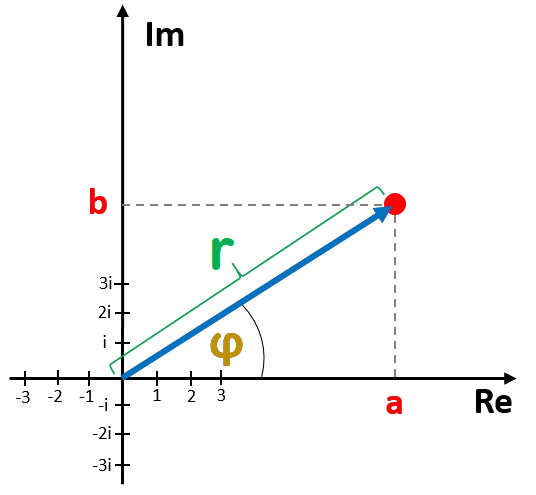# Complex Number to Polar Form Calculator

Created by Anna Szczepanek, PhD
Reviewed by Komal Rafay
Last updated: May 04, 2023

This complex number to polar form calculator is here to serve whenever you need to quickly convert a complex number to polar form. Let's briefly recall what the polar form is and how the conversion works so that you know how to do it by hand if you ever face such a challenge.

## What is the polar form of a complex number?

There are a few ways to represent a given complex number, and the polar form is one of them. You're most probably already familiar with the form z = a + bi, where a and b are the rectangular coordinates.

The polar form uses the fact that z can be identified by two numbers:

• The distance r from the origin of the plane (i.e., the point (0,0)) to z; and
• The angle φ between the horizontal axis and the radius connecting the origin and z.

We call r the modulus (or the magnitude) and φ the argument of z.

The trigonometric form of a complex number z reads

z = r × [cos(φ) + i × sin(φ)],

where:

• r is the modulus, i.e., the distance from (0,0) to z; and
• φ is the argument, i.e., the angle between the x-axis and the radius between (0,0) and z.Let us discuss how to convert from a rectangular form complex number to polar form.

## Conversion formula for the polar form

To understand how to convert from complex number a + bi to polar form, we need to recall the Pythagorean theorem and some basics of trigonometry.

Look at the figure above and note that

r² = a² + b²

as well as

tan(φ) = b / a.

The first formula tells us how to get r from a and b:

r = √(a² + b²).

Getting φ is slightly more complicated as it is trapped under the tangent function. We need to compute the inverse of the tangent function, that is, the arcus tangent (atan) function:

φ = atan2(b, a).

The mysterious atan2 function is defined simply as atan2(b, a) = arctan(b / a) if a > 0. But if a < 0, then atan(b / a) does not give us the right answer - we must correct it by ±π to end up in the correct quadrant of the plane. The definition of atan2(b, a) provides this correction. Formally, atan2(b, a) is equal to:

• atan(b / a) if a > 0;
• atan(b / a) + π if a < 0 ≤ b;
• atan(b / a) - π if a,b < 0;
• π/2 if a = 0 < b;
• -π/2 if b < 0 = a; and
• remains undefined if x = y = 0.

These are exactly the formulas behind Omni's polar form converter.

🙋 The best way to deal with atan is to use Omni's arcus tangent calculator. Or, in the particular context of converting complex numbers to polar form, the best way is, of course, to use our complex number to polar form calculator :)

## How to use this complex number to polar form calculator

This tool is really straightforward to use: given a complex number in its rectangular form, so the a + bi form, you need to input a and b into their respective fields.

Our complex number to polar form calculator will immediately display the magnitude r and phase (argument) φ of your number, so that you can write down the polar form r × exp(iφ).

As complex numbers are an essential tool in math and science, the Omni team built a rich collection of tools related to this topic:

## FAQ

### How do I convert a complex number to polar form?

To convert a + bi to polar form, follow these steps:

1. Compute r = √(a² + b²). This is the magnitude (modulus) of your number.
2. Compute φ = atan(b / a). If needed, correct by ±π to get into the correct quadrant. This is the argument (phase) of your number.
3. Write down your number as r × exp(iφ).
4. If you have trouble computing tan-1, do not hesitate to use an online arcus tangent calculator.

### What is the polar form of i?

i = exp(i π/2). To see why this is the right answer, observe that the distance between i and the points (0,0) is equal to 1. Hence, the modulus of i is 1. As for the argument, it equals 90° = π/2, because the angle between the x-axis and i is the right angle.

### What is the polar form of 0?

0 is the only complex number that does not have a unique polar representation: while its modulus (magnitude) is obviously 0, the argument (phase) can be anything. Often it is, by convention, taken as 0` as well.

Anna Szczepanek, PhD
Rectangular form a + bi
Real part (a)
Imaginary part (b)
Polar form r × exp(iφ)
Magnitude (r)
Phase (φ)
deg
People also viewed…

### Arcus tangent

With this arcus tangent calculator you'll quickly find the inverse of a tangent.

### Black Friday

How to get best deals on Black Friday? The struggle is real, let us help you with this Black Friday calculator!

### Car vs. Bike

Everyone knows biking is fantastic, but only this Car vs. Bike Calculator turns biking hours into trees! 🌳

### Matrix rank

The matrix rank calculator is an easy-to-use tool to calculate the rank of any matrix with up to four rows or columns.